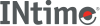﻿ logbINtime SDK Help
logb

Computes the exponent of `x`, which is the integral part of logr |`x`|, as a signed floating-point value, for non-zero `x`, where `r` is the radix of the machine's floating-point arithmetic, which is the value of FLT_RADIX defined in the <float.h> header.

If `x` is subnormal it is treated as though it were normalized; thus for finite positive `x`:

```1 <= x * FLT_RADIX-logb(x) < FLT_RADIX
```
```#include <math.h>

double logb (double x);
```

#### Return Values

Upon successful completion, these functions shall return the exponent of `x`.

If `x` is +/-0, a pole error shall occur and logb() shall return -HUGE_VAL.

If `x` is NaN, a NaN shall be returned.

If `x` is +/-Inf, +Inf shall be returned.

#### Requirements

Versions Defined in Include Link to
INtime 3.0 intime/rt/include/math.h math.h clib.lib